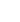15min:
THE PERMANENT ELECTRIC DIPOLE MOMENT OF CaD \setcounterfootnote1..

JINHAI CHEN, TIMOTHY C. STEIMLE, Department of Chemistry and Biochemistry, Arizona State University, Tempe, AZ 85287.

In 2004 we determined from the analysis of the Stark effect in the A2- X2+ (0,0) band of system the magnitude of the permanent electric dipole moment, \vertµ\vert, of CaH to be 2.94(16) D and 2.372(12)D for the X22 (v=0) and A2(v=0) states,respectively. The numbers in parenthesis are 1error estimates. Recently, two groups have performed high-level, all-electron, ab initio calculation and predicted µ values of 2.623 D and 2.617 D for the X2+(v=0) state. Although inside a 2error estimate, these values are somewhat smaller than the experimentally observed value. In an effort to improve the precision of \vertµ\vert, the Stark effect in the B2+ - X2+ (0,0) band system of CaD has been recorded and analyzed. As expected, the Stark effect is about four times larger in CaD compared to CaH. The analysis gives \vertµ\vert of 2.633(27) D and 2.018(33) D for the X2+(v=0) and B2+(v=0) state, respectively. A discussion of the isotopic dependence of µ and experimental systematic errors will be given. The trend in µ for the X2+, A2and B2+ is modeled using a simple molecular orbital picture.